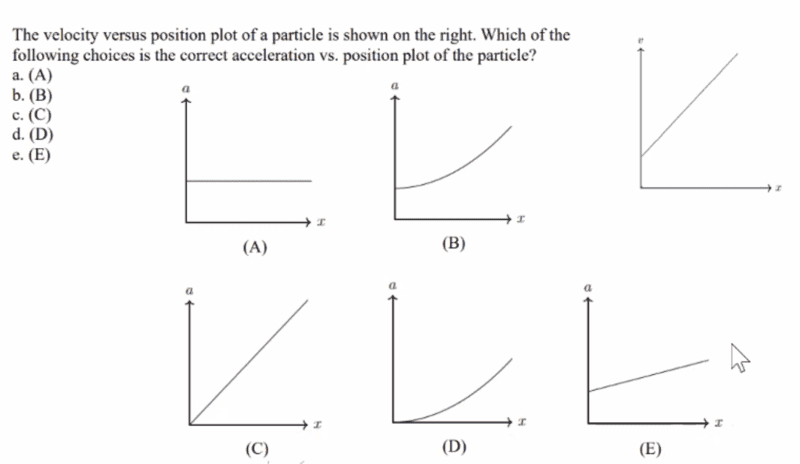# Finding acceleration from Velocity vs Position graph

iceninja3
Homework Statement:
The velocity versus position plot of a particle is shown on the right. Which of the following choices is the correct acceleration vs position plot of the particle (picture attached)?
Relevant Equations:
dv/dt = a
dx/dt = vThe answer is E. I was initially very confused as to why the answer was not A but realized that the graph was velocity vs position (rather than velocity vs time) which means I can't simply take the derivative of the given graph.

One thing I tried was writing out the equation first(c being a constant):
v = cx
*differentiating both sides with respect to time*
a = c•(v) = (c^2)•x
So a constant times x which rules out choices B and D.

Having recognized that I am still not sure how to move forward to prove to myself the answer is E. Any hints?

•berkeman

Gold Member
Think about the velocity as a function of ##x## as a linear function:

$$v(x) = b + mx$$

You are going to use the chain rule to get ##\frac{dv}{ dt}##

•iceninja3
iceninja3
Think about the velocity as a function of ##x## as a linear function:

$$v(x) = b + mx$$

You are going to use the chain rule to get ##\frac{dv}{ dt}##
Ohhh I see. So if I do the chain rule I would get:
a = mv and then substituting x back:
a = m(mx+b)
a = m^2x + mb
m^2x + mb rules out B and D because x is linear. And rules out C because the y-intercept is nonzero. And rules out A because the slope is nonzero.

Thank you so much!

•erobz and SammyS